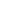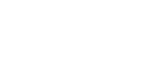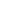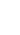Previous Next

## Tips on estimating sample size

User Rating: 5 / 5### What is the sample size?

The sample size is a frequently-used term in statistics and market research, and one that inevitably comes up whenever you’re surveying a large population of respondents. It relates to the way research is conducted on large populations. So what is sampling, and why does sample size matter?

When you survey a large population of respondents, you’re interested in the entire group, but it’s not really possible to get answers or results from absolutely everyone. So you take a random sample of individuals that represents the population as a whole.

The size of the sample is very important for getting accurate, statistically significant results, and running your study successfully.

• If your sample is too small, you may include a disproportionate number of individuals which are outliers and anomalies. These skew the results and you don’t get a fair picture of the whole population.
• If the sample is too big, the whole study becomes complex, expensive, and time-consuming to run, and although the results are more accurate, the benefits don’t outweigh the costs

If you’ve already worked out your variables you can get to the right sample size quickly with the online sample size calculator below:

1. Confidence Level:
2. Population Size:
3. The margin of Error:

### Learn how to determine the sample size

To choose the correct sample size, you need to consider a few different factors that affect your research and gain a basic understanding of the statistics involved. You’ll then be able to use a sample size formula to bring everything together and sample confidently, knowing that there is a high probability that your survey is statistically accurate.

The steps that follow are suitable for finding a sample size for quantitative data

• #### Stage 1: Consider your sample size variables

Before you can calculate a sample size, you need to determine a few things about the target population and the level of accuracy you need:

1.  Population size: How many people are you talking about in total? To find this out, you need to be clear about who does and doesn’t fit into your group. For example, if you want to know about dog owners, you’ll include everyone who has at some point owned at least one dog. (You may include or exclude those who owned a dog in the past, depending on your research goals.) Don’t worry if you’re unable to calculate the exact number. It’s common to have an unknown number or an estimated range.
2. The margin of error (confidence interval): Errors are inevitable – the question is how much error you’ll allow. The margin of error, AKA confidence interval, is expressed in terms of mean numbers. You can set how much difference you’ll allow between the mean number of your sample and the mean number of your population. If you’ve ever seen a political poll on the news, you’ve seen a confidence interval and how it’s expressed. It will look something like this: “68% of voters said yes to Proposition Z, with a margin of error of +/- 5%.”
3. Confidence level: This is a separate step to the similarly-named confidence interval in step 2. It deals with how confident you want to be that the actual mean falls within your margin of error. The most common confidence intervals are 90% confident, 95% confident, and 99% confident.
4. Proportions (p,q): Probability that you will pick a right person or well informed about the topic or the hypothesis you are researching on. P is the probability of success and q=1-p is the probability of failure to pick the right informed respondent. Many researchers advise taking p=50% and q=50%, however, if you are sure of your sampled population you can approximate to p=80% and q=80%. A good example for community developments projects, in most cases beneficiaries can not leave their communities by at least 20%

• #### Stage 2: Calculate sample size

Now that you’ve got answers for steps 1 – 4, you’re ready to calculate the sample size you need. This can be done using an online sample size calculator or with paper and pencil.

1. Find your Z-score: Next, you need to turn your confidence level into a Z-score. Here are the Z-scores for the most common confidence levels:
• 90% – Z Score = 1.645
• 95% – Z Score = 1.96
• 99% – Z Score = 2.576. If you chose a different confidence level, use this Z-score table (a resource owned and hosted by SJSU.edu) to find your score.
2. Use the Cochran's sample size formula: Plug in your Z-score, standard of deviation, and confidence interval into the sample size calculator or use this sample size formula to work it out yourself:Where N= Population, Marginal of Error (MoE) or e = 5% also called Sample error,  Zα/2 = a constant associated with the confidence level that is being used for this case we use the 95% confidence level (α=5%), Z=1.96. Here’s a worked example, assuming you chose a 95% confidence level, .5 standard deviation, and a margin of error (confidence interval) of +/- 5% for a population of N=100,000

Then  n=100,000 x (1.96)2 x 0.5 x 0.5) / ((100,000-1) x (.05)2 +(0.5 x 0.5 x (1.96)2))= 382.7 (approximated to 383).

In case when the Population of interest is Unkown, then use the formulaWe have a summary table for estimating the samples below. if you need the excel file subscribe to our newsletter and we will send via your emailToday76
Yesterday132
This week208
This month2947
Total53027

1
Online

2021-07-27

### What We Do

• General Research
• Market Research
• Project evaluation
• Capacity building
• Data management
• Design paperless data collection systems
• Selling Software

### Latest News

Purchase Software 29 March 2021
CSweb Server 29 March 2021
•